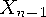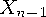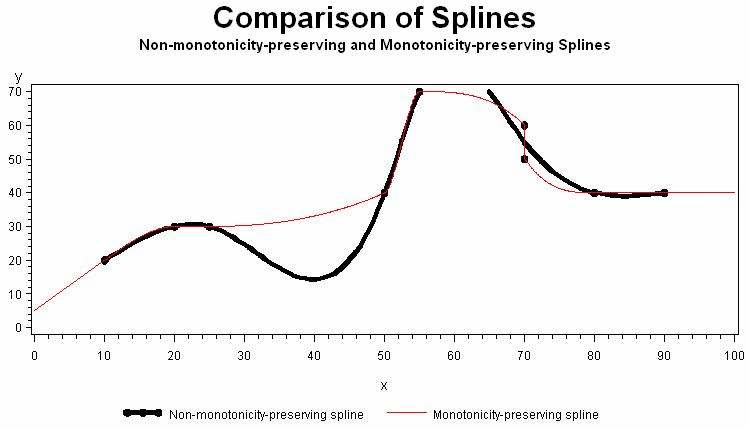Functions and CALL Routines

# MSPLINT Function

Returns the ordinate of a monotonicity-preserving interpolating spline.
 Category: Mathematical

## Syntax

 MSPLINT(X, n, X1 <, X2, ..., Xn>, Y1 <,Y2, ..., Yn> <, D1, Dn>)

### Arguments

X

is a numeric constant, variable, or expression that specifies the abscissa for which the ordinate of the spline is to be computed.

n

is a numeric constant, variable, or expression that specifies the number of knots. N must be a positive integer.

X1, ..., Xn

are numeric constants, variables, or expressions that specify the abscissas of the knots. These values must be non-missing and listed in nondecreasing order. Otherwise, the result is undefined. MSPLINT does not check the order of the X1 through Xn arguments.

Y1, ..., Yn

are numeric constants, variables, or expressions that specify the ordinates of the knots. The number of Y1 through Yn arguments must be the same as the number of X1 throughXn arguments.

D1, Dn

are optional numeric constants, variables, or expressions that specify the derivatives of the spline at X1 and Xn. These derivatives affect only abscissas that are less than X2 or greater than.

The MSPLINT function returns the ordinate of a monotonicity-preserving cubic interpolating spline for a single abscissa, X.

An interpolating spline is a function that passes through each point that is specified by the ordered pairs (X1, Y1), (X2, Y2), ..., (Xn, Yn). These points are called knots.

A spline preserves monotonicity if both of the following conditions are true:

• For any two or more consecutive knots with nondecreasing ordinates, all interpolated values within that interval are also nondecreasing.

• For any two or more consecutive knots with nonincreasing ordinates, all interpolated values within that interval are also nonincreasing.

However, if you specify values of D1 or Dn with the wrong sign, monotonicity will not be preserved for values that are less than X2 or greater than.

If the arguments D1 and Dn are omitted or missing, then the following actions occur:

• For n=1, MSPLINT returns Y1.

• For n=2, MSPLINT uses linear interpolation or extrapolation.

If the arguments D1 and Dn have non-missing values, or if n >= 3, then the following actions occur:

• If X < X1 or X > Xn, MSPLINT uses linear extrapolation.

• If X1 <= X <= Xn, MSPLINT uses cubic spline interpolation.

If two knots have equal abscissas but different ordinates, then the spline will be discontinuous at that abscissa. If two knots have equal abscissas and equal ordinates, then the spline will be continuous at that abscissa, but the first derivative will usually be discontinuous at that abscissa. Otherwise, the spline is continuous and has a continuous first derivative.

If X is missing, or if any other arguments required to compute the result are missing, then MSPLINT returns a missing value. MSPLINT does not check all of the arguments for missing values. Because the arguments D1 and Dn are optional, and they are not required to compute the result, if one or both are missing and no errors occur, then MSPLINT returns a non-missing result.

The following is an example of the MSPLINT function.

```data msplint;
do x=0 to 100 by .1;
msplint=msplint(x, 9,
10, 20, 25, 50, 55, 70, 70, 80, 90,
20, 30, 30, 40, 70, 60, 50, 40, 40);
output;
end;
run;

data knots;
input x y;
datalines;
10 20
20 30
25 30
50 40
55 70
70 60
70 50
80 40
90 40
;

data plot;
merge knots msplint;
by x;
run;

title "Comparison of Splines";
title2 "Non-monotonicity-preserving and Monotonicity-preserving
Splines";
legend1 value=('Non-monotonicity-preserving spline'
'Monotonicity-preserving spline') label=none;
symbol1 value=dot  interpol=spline color=black width=5;
symbol2 value=none interpol=join   color=red;
proc gplot data=plot;
plot y*x=1 msplint*x=2/overlay legend=legend1;
run;
quit; ```Fritsch, F. N., and J. Butland. 1984. "A method for constructing local monotone piecewise cubic interpolants." Siam Journal of Scientific and Statistical Computing 5:2, 300-304.Previous Page | Next Page | Top of Page